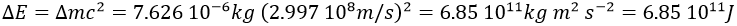# Chapter 1b: Atomic theory and nuclear reactions

Atomic theory

At the end of the XIX, it was known that the elements were made of one atom, unbreakable and different for each element. The masses of the atoms were known for several elements but their composition was still a mystery.

Michaël Faraday discovered that atoms were in fact composed of charged species, even if they are electrically neutral. His discovery was the result of an experiment in which a current passes through silver electrodes sunk in a solution containing silver (AgNO3). When the current passes, the mass of the electrode increased significantly. The silver ions in the solutions reacted with the electrons from the current to form solid silver gathering on the electrode.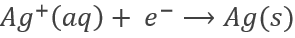This reaction showed that atoms contain positively charged elements, and therefore negatively charged species to neutralise the charge of the atom.

Joshep John Thompson proved the existence of electrons in 1897 during his works on cathodic tubes. Those tubes only contain void, a cathode and an anode. If the cathode is heated, a current is detected between the electrodes. The heating determines the kinetic energy transmitted to the atom of the cathode: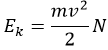While the charge e of the electrons is given by the current: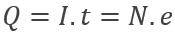Applying a magnetic field H on the cathodic tube, if only a small gap allowed electrons to reach the anode, no current was observed: the electron beam deviates from its normal trajectory depending on the ratio mass/charge. The walls of the gap were covered by ZnS, a fluorescent species to detect the deflection of the beam (radius of deflection r):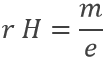As a result, the ratio e/m of an electron was determined: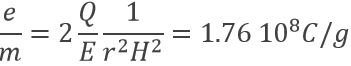J.J. Thompson imagined a model of a spherical atom wherein a sea of charged species are moving (the plum pudding model).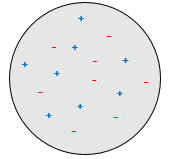The charge of the electron was determined by Robert Andrews Millikan. He beamed RX on droplets of oil between two horizontal electrodes. The charged droplets are subjected to several forces: their own weight, the electrostatic force and the friction of the air (air has a given viscosity).

The weight of a droplet is given by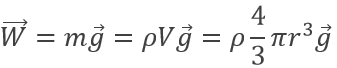m being the mass of a given droplet, equal to its density multiplied by its volume, and g is the gravity. The electrostatic force is given by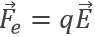where E is the electric field and q is the charge. The friction is given by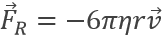where η is the air viscosity and v is the speed of the droplet. In absence of electric field, the droplet should fall at a speed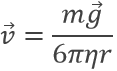resulting from the equations of W and FR. However in the electric field E, the speed of a droplet is affected: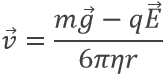Except q, all the terms of the right side of the equation are known and the speed of droplets was experimentally determined: a droplet is observed through a microscope to measure the time it takes to travel a given distance.

The result of the experiment was that the charge q was always a multiple of 1.602 10-19C, some droplets being several times charged.

The mass of an electron could thus be determined as well: me=9.11 10-31kg.

Despite his brilliant experiment, the plum pudding model of J.J Thompson was not correct and got refuted by Ernest Rutherford (who actually has been one of his student), the pioneer of the nuclear chemistry. Rutherford studied the emission of α particles from Uranium. α particles  are the equivalent of the nucleus of Helium atoms: 2 neutrons and 2 protons: He2+. α particles were beamed towards a thin foil of gold. Considering the model of Thompson, all of the radiation should have passed through the gold foil. 99% of it passed, and it was not due to an experimental error. Some of the α particles were deflected in all directions. He concluded that the 1% deflected on a solid aggregation with an intense positive charge and composing the majority of the mass of the element. The rest of the volume of an atom being filled by empty space and a cloud of electrons. The size of an atom is about 1 angström (1Å)=10-10m of diameter and the size of a nucleus is 10-15m.

Rutherford was also the first to transmute an element into another one. He did that by bombarding pure nitrogen by α particles and obtained oxygen and hydrogen nuclei (protons were not yet known). He assumed that hydrogen nuclei are part of the solid nucleus of the atoms. From now, atoms were no more unbreakable.

Later, Rutherford theorized the existence of neutrons to keep the positively charged nucleus in one piece, reducing the repelling of protons and giving a cohesion energy to the nucleus. The bigger the atom, the more neutrons are needed (in proportion with protons).

This energy of cohesion is actually enormous. For example Oxygen is made of 8 neutrons and 8 protons. But the mass of the atom of oxygen is smaller than the addition of the mass of the separated protons and neutrons: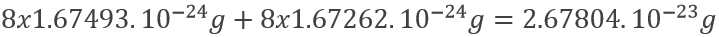The mass of an oxygen atom is 2.65535.10-23g. The difference is about 2.269.10-25g by atom. As E=mc2, we obtain for one mole an energy of cohesion of 1.23.1013J/mol of oxygen. For comparison, a typical chemical reaction is ~105J/mol. There is thus no surprise why nuclear reactions car produce so much energy.

Nuclear reactions

Nuclear chemistry is a very specific domain of chemistry. It is one domain where the Lavoisier rule does not apply: the elements are not conserved and mass can be converted into energy. Yet, several types of reactions can be sorted. But first, we will take a look at how to write the isotopes involved. We have seen that each chemical elements is an atom with a specific composition of protons, electrons and neutrons. This composition gives the sepcific properties of each element. However, there can be several forms for many elements. Those forms are the isotopes. They differ by their number of neutrons, the number of electrons and of protons being equal and fixed for every element. The chemical properties of isotopes are almost identical (because given by the electrons), but some physical properties can be different between isotopes of the same element. The speed of reaction and the ebullition temperature are two examples of properties that change depending of the isotope. The isotope 238 of Uranium is written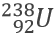U is the symbol of the element, the mass is written at the top left of the element and its atomic number Z is written at the bottom left. The atomic number can be skipped. The proportions the isotopes of a single element are not equal. For instance, the carbon has 2 stable isotopes: 12C and 13C with a proportion of 98.93% and 1.07%. 14C is an isotope of the carbon but it is not stable: it decays over time. Historians use this property to date ancient items or bodies.

1. Production of α particles

Remember that an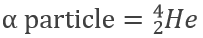The decay of Uranium 238 is an example of reaction producing α particles: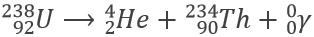The total value of the top number is conserved during the reaction. The same is true for the bottom number. The γ product is gamma radiation, produced by radioactive decays because the formed nucleus is generally in an exited mode. To come back to its base state, energy is thus freed in the form of an electromagnetic radiation

1. Production of β particles

The β particles are small charged species emitted during some nuclear reactions. The Thorium 234 obtained previously can produce this kind of particles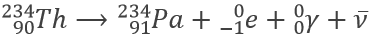The mass of the element did not change during this reaction. However, the element changed from Thorium to Protactinium. One neutron became a proton. The β particles produced during this reaction is an electron rejected from the neutron to become a proton.

An antineutrino is also generated. A nucleus has a given spin depending on its charge: it turns on itself in a given direction. Electrons also have a spin. During the nuclear reaction from above, the charge of the nucleus changed and an antineutrino is liberated to obtain the correct spin.

A second β particle can be obtained, for example during the decay of Sodium 22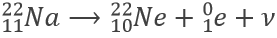The β particle is not an electron but a positron. A neutrino is also obtained during this reaction. Neutrinos and antineutrinos are radiations that can go through everything.

Electrons and positrons can neutralize to produce a gamma radiation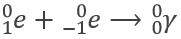1. Fission

Fission is done by bombarding an isotope with neutrons. In nuclear plants, fission is done on Uranium 235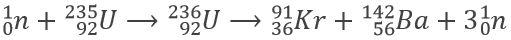There is more neutrons produced by the reaction than needed to launch it. This reaction can thus start again as long as there is Uranium 235 in the reactor. Another way to stop the reaction is to trap the neutrons with another isotope.

1. Fusion

Fusion is done by merging two isotopes. For example, two isotopes of Hydrogen can produce Helium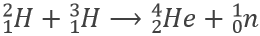Another way to obtain Helium is to bombard Hydrogen with electrons.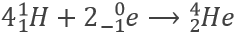It is also a fusion reaction.

The elements used in fusion and in fission are different. The energy of cohesion is different for each element and we can observe a maximum of energy of cohesion/nucleon (proton+neutron) for 56Fe. Fusion is performed on elements of lower mass up to the 56Fe. Atoms gain energy of cohesion during fusion. On the other side, elements of higher mass lose cohesion energy and mass up to the 56Fe.

Half-life time

Radioactive elements do not stay active forever. The radioactivity decreases over time proportionally at the number or particles.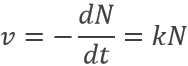where v is the speed of decomposition, N the number of radioactive particles and k is a speed constant depending on the isotope.

Because it depends on the number of particles, the speed decreases over time. We can integer the speed equation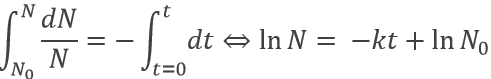The half-life time is the time needed to decrease the population of a radioactive element by half: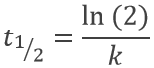This time does only depend on the isotope and does not depend on the population of the isotope.

Exercises

1.  Complete these nuclear reactions: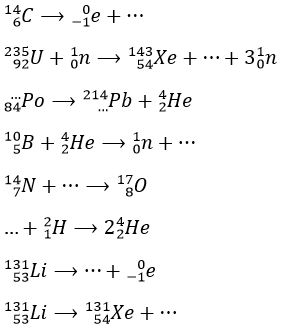2.  A piece of manuscript has been analysed for dating. It has been found that the ratio 14C/12C of the manuscript is equal to 0.802 times the value of a plant of today. Given that the half-life time of 14C is 5720years, what is the age of the manuscript?

3.  How much energy is generated by the fusion of 1.2g of deuterium (D or 21H)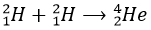given that the masses of the two species are MD=2.0141g/mol and MHe=4.0026g/mol?

1.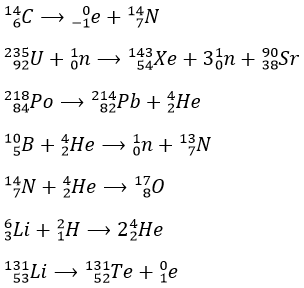2.  The half-life time of a radioactive element defines the time it takes to the element to decrease its population by half. The general formula to calculate the population of an isotope is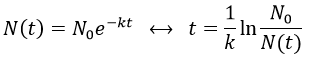The half-life time is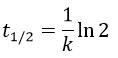It allows us to determine the value of k: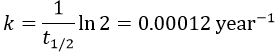The ratio 14C/12C that we have in the wording is the reverse of N0/N(t). Now that we know the value of k, we can thus find the age of the manuscripts with the equation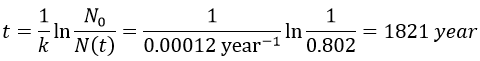3.  In 1.2g of deuterium, there is 1.2g/2.0141g/mol=0.5958mol. We need two deuterium to form one atom of helium, so the amount of helium after the reaction is 0.2979mol.

The energy ΔE generated by the reaction is proportional to the mass Δm lost during the reaction. This mass lost for each produced mole of He is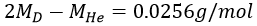Thus Δm=0.007626g for 1.2g of deuterium. The energy generated is given by